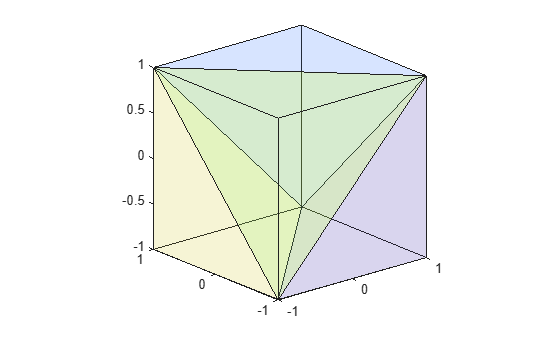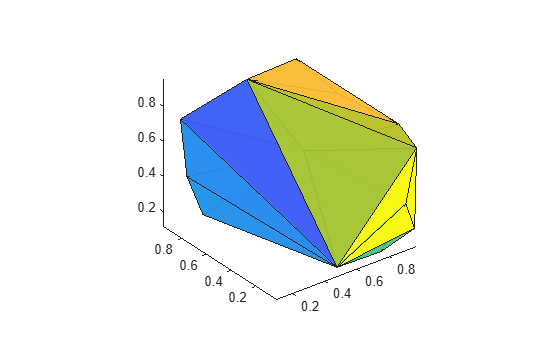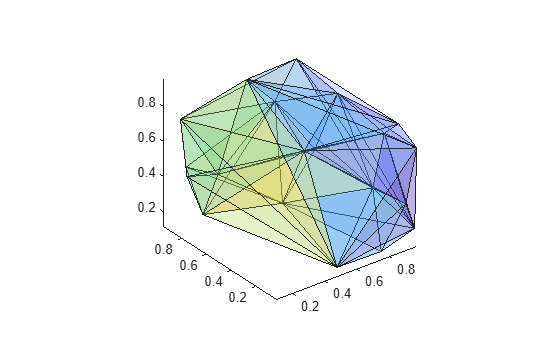# tetramesh

Tetrahedron mesh plot

## Syntax

``tetramesh(T,X)``
``tetramesh(TR)``
``tetramesh(___,c)``
``tetramesh(___,Name,Value)``
``h = tetramesh(___)``

## Description

example

````tetramesh(T,X)` plots the 3-D tetrahedron mesh defined by the tetrahedron connectivity matrix `T` and the points `X`.`T` is an `m`-by-`4` matrix that specifies the vertices of `m` tetrahedra. `X` is an `n`-by-`3` matrix that specifies the Cartesian coordinates of `n` points. Each row of `T` contains indices into `X` that specify the 4 vertices of a tetrahedron.```

example

````tetramesh(TR)` plots the tetrahedron mesh defined by a 3-D `triangulation` or `delaunayTriangulation` object.```

example

````tetramesh(___,c)` also specifies the face color of each tetrahedron using a vector of colormap indices.```

example

````tetramesh(___,Name,Value)` specifies one or more properties of the mesh plot using name-value arguments. For example, `'LineWidth',2` sets the edge width to `2` points.```
````h = tetramesh(___)` returns a vector of tetrahedron handles. Each handle of `h` is a `patch` object used to create the mesh plot of each tetrahedron. Use `h(i)` to query and modify properties of the plot of each tetrahedron. For more information, see Patch Properties.```

## Examples

collapse all

Create 8 points that represent the corners of a cube in Cartesian coordinates.

```d = [-1 1]; [x,y,z] = meshgrid(d); X = [x(:) y(:) z(:)]```
```X = 8×3 -1 -1 -1 -1 1 -1 1 -1 -1 1 1 -1 -1 -1 1 -1 1 1 1 -1 1 1 1 1 ```

Specify a tetrahedron connectivity matrix where each row contains the indices into `X` that specify the 4 vertices of a tetrahedron. For instance, the first row describes a tetrahedron with vertices consisting of the first, third, fourth, and seventh points.

```T = [1 3 4 7; 4 6 7 8; 1 5 6 7; 1 2 4 6; 1 4 6 7]```
```T = 5×4 1 3 4 7 4 6 7 8 1 5 6 7 1 2 4 6 1 4 6 7 ```

Use `tetramesh` to plot the 5 tetrahedra that form the cube.

```tetramesh(T,X); view(-40,20)```To see into the cube, plot it again with more transparent faces.

```tetramesh(T,X,'FaceAlpha',0.1); view(-40,20)```Create a 3-D `delaunayTriangulation` object for 20 random points.

```rng('default'); x = rand([20 1]); y = rand([20 1]); z = rand([20 1]); DT = delaunayTriangulation(x,y,z)```
```DT = delaunayTriangulation with properties: Points: [20x3 double] ConnectivityList: [53x4 double] Constraints: [] ```

Plot the tetrahedron mesh.

`tetramesh(DT)`Change the color of the tetrahedra. Plot it again with the new colors and with more transparent faces.

`tetramesh(DT,106:-2:1,'FaceAlpha',0.1)`## Input Arguments

collapse all

Tetrahedron connectivity, specified as a 4-column matrix. Each row of `T` contains indices into `X` that specify the 4 vertices of a tetrahedron.

Coordinates of points, specified as a 3-column matrix.

3-D triangulation object, specified as a `triangulation` or `delaunayTriangulation` object.

Face color, specified as a vector of colormap indices of the same size as the number of tetrahedra. The tetrahedron colors are defined by the vector `c`, which is used as indices into the current colormap.

To customize the color scheme, use the `colormap` function. For additional control over the surface coloring, use the `'EdgeColor'` and `'FaceColor'` name-value arguments.

### Name-Value Arguments

Specify optional pairs of arguments as `Name1=Value1,...,NameN=ValueN`, where `Name` is the argument name and `Value` is the corresponding value. Name-value arguments must appear after other arguments, but the order of the pairs does not matter.

Before R2021a, use commas to separate each name and value, and enclose `Name` in quotes.

Example: `tetramesh(TO,'LineStyle',':')`

The properties listed here are only a subset. For a complete list, see Patch Properties.

Face color, specified as the comma-separated pair consisting of `'FaceColor'` and a color name, an RGB triplet, or `'none'`.

An RGB triplet is a three-element row vector whose elements specify the intensities of the red, green, and blue components of the color. The intensities must be in the range `[0,1]`; for example, `[0.4 0.6 0.7]`. This table lists the long and short color name options and the equivalent RGB triplet values.

Long NameShort NameRGB Triplet
`'yellow'``'y'``[1 1 0]`
`'magenta'``'m'``[1 0 1]`
`'cyan'``'c'``[0 1 1]`
`'red'``'r'``[1 0 0]`
`'green'``'g'``[0 1 0]`
`'blue'``'b'``[0 0 1]`
`'white'``'w'``[1 1 1]`
`'black'``'k'``[0 0 0]`

Face transparency, specified as the comma-separated pair consisting of `'FaceAlpha'` and a scalar in the range `[0,1]`. A value of 1 is opaque and 0 is completely transparent. Values between 0 and 1 are semitransparent.

Edge color, specified as the comma-separated pair consisting of `'EdgeColor'` and a color name, an RGB triplet, or `'none'`. The default color of `[0 0 0]` corresponds to black boundaries.

An RGB triplet is a three-element row vector whose elements specify the intensities of the red, green, and blue components of the color. The intensities must be in the range `[0,1]`; for example, ```[0.4 0.6 0.7]```. This table lists the long and short color name options and the equivalent RGB triplet values.

Long NameShort NameRGB Triplet
`'yellow'``'y'``[1 1 0]`
`'magenta'``'m'``[1 0 1]`
`'cyan'``'c'``[0 1 1]`
`'red'``'r'``[1 0 0]`
`'green'``'g'``[0 1 0]`
`'blue'``'b'``[0 0 1]`
`'white'``'w'``[1 1 1]`
`'black'``'k'``[0 0 0]`

Line width, specified as the comma-separated pair consisting of `'LineWidth'` and a positive numeric value in points.

Line style, specified as one of the options listed in this table.

Line StyleDescriptionResulting Line
`"-"`Solid line`"--"`Dashed line`":"`Dotted line`"-."`Dash-dotted line`"none"`No lineNo line

## Version History

Introduced before R2006a